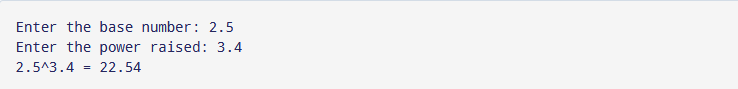## Example program for pow function in c

pow function c programming is use to computer the power of a given number to the function.

pow() c function takes two arguments first one is known as base and second one as power value and pow() c function return a base with the power raised to that base number.

## Syntax for pow() c

`[Mathematics] xy = pow(x, y) [In programming]`

pow c function defines under the math.h header file in c.

If you want to find the pow of any int and float number then convert the operator type into the double.

```int base = 3;
int power = 5;
pow(double(base), double(power));```

# Example for C pow() function

```#include <stdio.h>
#include <math.h>```program output for pow function in c